# RS Aggarwal Class 9 Solutions Chapter 11 - Circle Ex 11B (11.2)

## RS Aggarwal Class 9 Chapter 11 – Circle Ex 11B (11.2) Solutions Free PDF

We have provided here the solutions of RS Aggarwal Class 9 maths textbook prepared by a team of subject experts which are complete in all aspect delivering students with a clear understanding of the topics. The simple and understandable language of the solutions surely is going to help students in scoring higher marks in the exam. You will find the RS Aggarwal Class 9 solutions in properly arranged format to aid students in the best way possible.

These solutions cover the entire syllabus and provide step by step solutions to the questions asked in RS Aggarwal maths textbook in a concise and accurate manner. Scoring good marks is easy with the access of these Class 10 maths solutions of RS Aggarwal. The well-structured solutions of RS Aggarwal Class 9 Solutions Chapter 11 – Circle Ex 11B (11.2) help students in fetching good marks in the exam.

## Download PDF of RS Aggarwal Class 9 Solutions Chapter 11 – Circle Ex 11B (11.2)

Question 1: In the given figure, O is the centre of the circle and ∠ AOB = 70°  calculate the values of i) ∠ OCA  ii)∠ OAC

Sol.

(i) The angle subtended by an arc of a circle at the center is doubled the angle subtended by the arc at any point on the circumference.

∴ ∠ AOB = 2 × ∠ OCA

⇒ ∠ OCA = 70°/2 = 35° [∵ ∠ AOB =70°]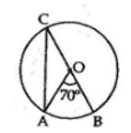(ii) The radius of the circle is

OA = OC

⇒ ∠ OAC = ∠ OCA [∵ base angles of an isosceles triangle are equal]

⇒ ∠ OAC = 35° [as ∠ OCA = 35°]

Question 2:  In the given figure , O is the centre of the circle . If ∠ PBC and ∠ B calculate the values of ∠ ADB.

Sol.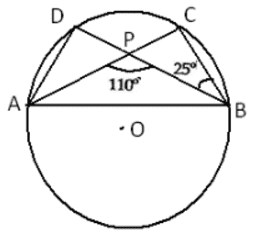It is clear that ∠ ACB = ∠ PCB

Consider the triangle Δ PCB

Applying the sum property, we have

∠ PCB = 180° – (∠ BPC + ∠ PCB)

= 180° – (180° – 110° + 25°)

∠ PCB = 180° – 95° = 85°

Angles in the same segment of a circle are equal.

∴ ∠ ADB = ∠ ACB = 85°

Question 3: In the given figure, O is the centre of the circle.If ∠ ABD = 35° and ∠ BAC = 70°, find ∠ ACB.

Sol.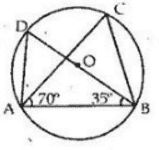It is clear that, BD is the diameter of the circle.

Also we know that, the angle in a semicircle is a right angle.

∴ ∠ BAD = 90°

Now consider the triangle Δ BAD

⇒   ∠ ADB = 180° – (∠ BAD + ∠ABD) [Angle sum property]

⇒      = 180° – (90° + 35°) [∠ BAD = 90° and ∠ABD= 35°]

⇒   ∠ ADB = 55°

Angles in the same segment of a circle are equal.

∴ ∠ACB = ∠ ADB = 55°

∴ ∠ACB = 55°

Question 4: In the given figure, O is the centre of the circle. If ∠ACB = 50°, Find ∠OAB .

Sol.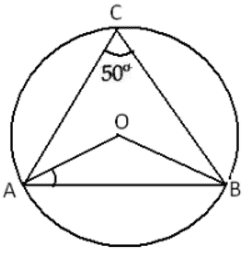The angle subtended by an arc of a circle at the center is doubled the angle subtended by the arc at any point on the circumference.

∴ ∠ AOB = 2 × ∠ ACB

= 2 × 50° [Given]

⇒ ∠AOB = 100° …….(1)

Now, in Δ OAB, we have

OA = OB [radius of the circle]

⇒ ∠ OAB = ∠ OBA [∵ base angles of an isosceles triangle are equal]

Thus we have

∠ OAB = ∠ OBA …….(2)

By angle sum property, we have

Now   ∠AOB + ∠ OAB + ∠ OBA = 180°

⇒ 100° + 2∠ OAB = 180°

⇒ 2∠ OAB = 180° – 100° [from (1) and (2)]

⇒ ∠ OAB = 80°/2 = 40°

∴ ∠ OAB = 40°

Question 5: In the given figure, ∠ABD = 54°  and ∠ABD = 43° . Calculate

i) ∠ACD

ii) ∠BDA

iii) ∠BDA

Sol.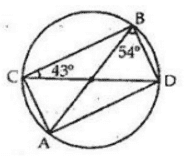(i) Angles in the same segment of a circle are equal.

∠ ABD and ∠ ACD are in the segment AD.

∴ ∠ ACD = ∠ ABD

= 54° [Given]

(ii) Angles in the same segment of a circle are equal.

∠ BAD and ∠ BCD are in the segment BD.

∴ ∠ BAD = ∠ BCD

= 43° [Given]

(iii) Consider the Δ ABD.

By angle sum property, we have

∠ BAD + ∠ADB + ∠ DBA = 180°

⇒ 43° + ∠ ADB + 54° = 180°

⇒ ∠ ADB = 180° -97° = 83°

⇒ ∠ BDA = 83°

Question 6: In the adjoining figure ,DE is the chord parallel to diameter AC of the circle with Centre O. If ∠ CBD = 60°, calculate ∠ CDE.

Sol.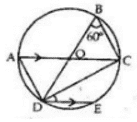Angles in the same segment of a circle are equal.

∴   ∠ CAD =∠ CBD = 60° [Given]

We know that an angle in a semi-circle is a right angle.

∴   ∠ ADC = 90° [angle in a semi-circle]

∴   ∠ ADC = 180° – (∠ ADC + ∠ CAD)

= 180° – (90° + 60°)

= 180° -150° =30°

∴   ∠ CDE = ∠ ADC =30°

Question 7: In the adjoining figure, O is the centre of the circle. Chord CD is parallel to diameter AB. If∠ ABC = 25°, calculate ∠ CED.

Sol.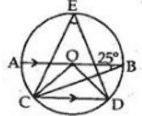Join CO and DO, ∠ BCD = ∠ ABC = 25° [alternate interior angles]

The angle subtended by an arc of a circle at the centre is double the angle subtended by the arc at any point on the circumference.

∴   ∠BOD = 2∠ BCD

= 50° [∠ BCD =25°]

Similarly,

∠AOC = 2∠ ABC

= 50°

AB is a straight line passing through the center.

∴    ∠AOC + ∠COD + ∠BOD = 180°

⇒ 50° + ∠ COD + 50° = 180°

⇒ ∠ COD = 180° – 100° = 80°

∴ ∠ CED = ½ ∠ COD

= 80°/2 = 40°

∴ ∠ CED = 40°

Question 8: In the given figure AB and CD are straight lines to the centre O of a circle .If ∠ AOC= 80° and ∠ CDE = 40° find

I – ∠ DCE

II- ∠ ABC

Sol.

(i) ∠ CED = 90°

In CED, We have

∠ CED + ∠ EDC + ∠ DCE =180°

⇒ 90° + 50° + ∠ DCE = 180°

∴ ∠ DCE = 180° -130°

∠ DCE = 50°   ….(1)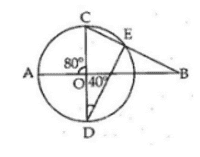(ii) ∠ AOC and ∠ BOC are linear Pair.

∴ ∠ BOC = (180° – 80°) = 100° ….(2)

∴ ∠ABC = 180° – (∠ BOC +∠ DCE)

= 180° – (100° + 50°) [from (1) and (2)]

= 180° – 150° = 30°

Question 9: In the given figure, O is the centre of the circle , ∠ AOB = 40° and ∠ BDC = 100° , find ∠ OBC = 60°

Sol.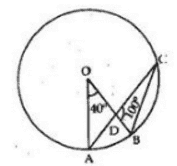The angle subtended by an arc of a circle at the centre is double the angle subtended by the arc at any point on the circumference.

∴   ∠AOB = 2∠ ACB

⇒    = 2∠ ACB [∵ ∠ ACB = ∠ DCB]

$\Rightarrow \angle DCB = \frac{1}{2}\angle AOB$

= $\left ( \frac{1}{2}\times 40 \right )$ = 20°

Consider the Δ DBC;

By angle sum property, we have

∠ BDC + ∠DCB + ∠ DBC = 180°

⇒ 100° + 20° + ∠DCB = 180°

⇒ ∠ DCB = 180° – 120° = 60°

⇒ ∠ OBC = ∠ DBC = 60°

∴ ∠ OBC = 60°

Question 10: In the adjoining figure ,choprd AC and BD of a circle with centre O , intersect at right angle at E . If  ∠ OAB = 25°, Calculate ∠ EBC

Sol.

Join OB,

∴ OA = OB [Radius]

∴ ∠ OBA = ∠ OAB = 25° [base angles are equal in isosceles triangle]

Now in Δ OAB, WE have

⇒ ∠OAB + ∠ OBA + ∠ AOB = 180°

⇒  25 + 25 + ∠AOB =180°

⇒  ∠AOB = 180° – 50° = 130°

The angle subtended by an arc of a circle at the centre is double the angle subtended by the arc at any point on the circumference.

∴ ∠ AOB = 2∠ ACB

⇒  ∠ACB = ½ ∠ AOB = ½ × 130° = 65°

⇒  ∠ECB = 65°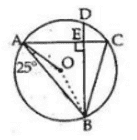In rt  angled Δ BEC,

We know that sum of three angles in a triangle is 180°.

⇒  ∠EBC + ∠BEC +∠ECB = 180°

⇒ ∠EBC + 90° + 65° = 180°

⇒ ∠EBC = 180° – 155° =25°

∴ ∠EBC =25°

Question 11:In the fgiven figure , O is the centre of the circle in which ∠ OAB = 20° and ∠ OCB = 55° . Find

1. ∠ BOC
2. ∠ AOC

Sol.

OB = OC [Radius]

⇒ ∠ OBC = ∠ OCB =55° [base angles are equal in isosceles triangle]

Consider the triangle Δ BOC.

By angle sum property, we have

∠ BOC = 180° – (∠ OCB + ∠ OBC)

= 180° – (55° + 55°)

= 180° – 110° = 70°

∴ ∠ BOC = 70°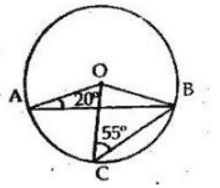Again, OA = OB

⇒ ∠ OBA = ∠ OAB =20° [base angles are equal in isosceles triangle]

Consider the triangle Δ AOB.

By angle sum property, we have

⇒ ∠ AOB = 180° – (∠ OAB + ∠ OBA)

= 180° – (20° + 20°)

= 180° – 40° = 140°

∴ ∠ AOC = ∠ AOB + ∠ BOC

= 140° – 70° = 70°

∴ ∠ AOC = 70°

Question 12: In the given figure , ∠ OAB = 25°. Show that BC is equal to the radius of the circumcircle of Δ ABC whose centre is O.

Sol.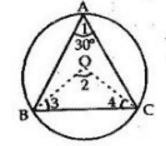Join OB and OC.

The angle subtended by an arc of a circle at the centre is double the angle subtended by the arc at any point on the circumference.

∴ ∠ BOC = 2∠ BAC

= 2 × 30° [∴∠ BAC = 30°]

= 60° …….(1)

Now Consider the triangle Δ BOC.

OB = OC [Radii]

⇒ ∠OBC = ∠ OCB …….(2)   [base angles are equal in isosceles triangle]

Now in Δ BOC, we have

⇒  ∠BOC + ∠OBC +∠OCB = 180°

⇒  60° + ∠OBC +∠OCB = 180° [from (1) and (2)]

⇒  2∠ OBC = 180° – 60° = 120°

⇒ ∠ OBC = 120°/2 = 60°

⇒ ∠ OBC = 60° [from (2)]

Thus, we have

∠BOC = ∠OBC = ∠OCB = 60°

So, BOC is an equilateral triangle

⇒ OB = OC = BC

∴   BC is the radius of the circumference.’

### Key Features of RS Aggarwal Class 9 Chapter 11 – Circle Ex 11B (11.2)

• It is the best revision study material for Class 9 students.
• It provides different methods to solve tricky and difficult questions.
• It helps you analyze your overall performance, speed and accuracy.
• It boosts your confidence while attempting the final paper.